HOME COURSES PREVIEW REVIEW ABOUT CONTACTTOLL-FREE INFO & ORDERING: M-F: 9am-5pm (PST): (877) RAPID-10

 24/7 Technical Support:

 Quick Search: Keywords:

 Rapid Learning Member Area: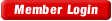Note: If you are a legacy user of chemistry24 members, please request a new login access to the premium server with your full name and old login email via vip@rapidlearningcenter.com

 Rapid Courses Catalog : Physics in 24 Hours Chemistry in 24 Hours Biology in 24 Hours Mathematics in 24 Hours

Weekly Physics Tips:
Want to become a top gun in your class? How about study less yet score high? Sign up this Physics Survival Weekly to learn how. Designed specifically for students who are taking physics, this free newsletter will show you how to survive and excel in class! Weekly topics include:
• How to Study Physics Effectively
• How to Read Physics Textbooks Easily
• How to Solve Physics Problems Systematically
• How to Score High on Physics Exams Strategically
• How to Master Physics Rapidly
Each week, you will receive study tips on the topics above and visual tutorial or study template to enhance your physics learning. Enter your name and email below to subscribe free:

 Your Name * Email *

 Physics Study Lounge These study sheets are for quick review on the subjects. Refer to our rapid courses for comprehensive review.     - Basic Skills in Physics     - Basic Math for Physics     - How to Solve Physics Problems     - Newton's Laws Study Guide     - ElectroMagnetism Quick Review     - Atomic Physics At-A-Glance     - Core Concepts in Nuclear Physics     - Special Relativity Overview

 Other Related Sites
 Note: For course links to launch, disable popup blockers or hold the ctrl key while clicking the link.Home » College Calculus

Separable Differential Equations

 Topic Review on "Title": Differential Equations: A differential equation is any equation that contains one or more derivatives. Separable differential equations: A separable differential equation is a differential equation of the formwhere forHomogeneous differential equations: A homogeneous differential equation is a differential equation of the form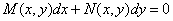where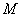and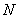are of the same degree.

Rapid Study Kit for "Title":
 Flash Movie Flash Game Flash Card Core Concept Tutorial Problem Solving Drill Review Cheat Sheet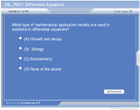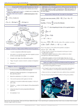"Title" Tutorial Summary : Separable differential equations are introduced with the introduction of differential equations and homogeneous equations. Differential equations are solved using calculus techniques. Examples are provided in this tutorial to present the idea of differential equations and their applications. Steps to solving differential equations are introduced with the help of examples. Homogeneous equations are exhibited with the aid of visual representation and graphs. Ideas to look forward in future courses are mentioned here with the help of examples.

 Tutorial Features: Specific Tutorial Features: True and answer questions are presented to evaluate the most important concepts in this tutorial. Step by step detailed examples showing all aspects of how to solve homogeneous and separable differential equations. Series Features: Concept map showing inter-connections of new concepts in this tutorial and those previously introduced. Definition slides introduce terms as they are needed. Visual representation of concepts Animated examples—worked out step by step A concise summary is given at the conclusion of the tutorial.

 "Title" Topic List: `Differential equations The definition of differential equation Examples of differential equationsSeparable differential equations Definition of separable differential equationsHomogeneous differential equations Definition of homogeneous differential equations`

See all 24 lessons in college calculus, including concept tutorials, problem drills and cheat sheets:
Teach Yourself College Calculus Visually in 24 Hours